# Essays on applied economics

 Lemma: The symmetric equilibrium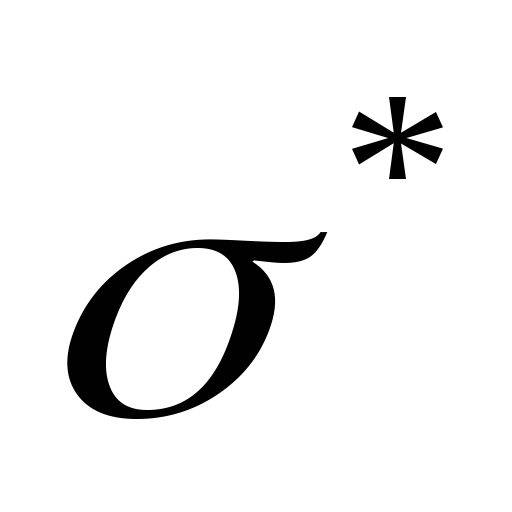is defined as follows: if the buyer is the current high bidder at any auction, or if the buyer’s valuation is less than or equal to the lowest standing bid, the buyer should pass; otherwise, if there is a unique lowest standing bid, the buyer should submit a bid with the seller offering the lowest standing bid. The bid should be equal to the smallest valuation that exceeds this lowest standing bid; otherwise, let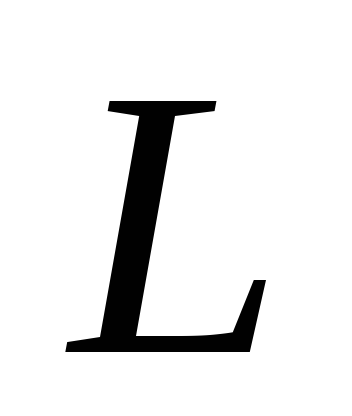be the set of sellers who have the lowest standing bid. Let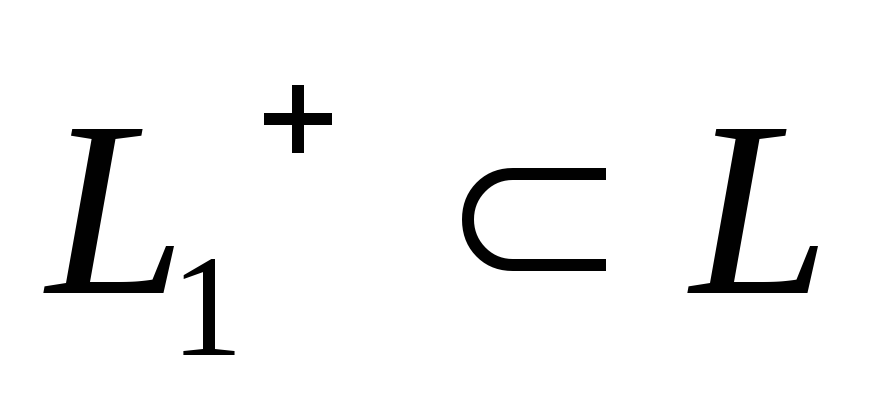be the subset of sellers inwhose current high bidder submitted his bid while the standing bid was strictly below its current level; let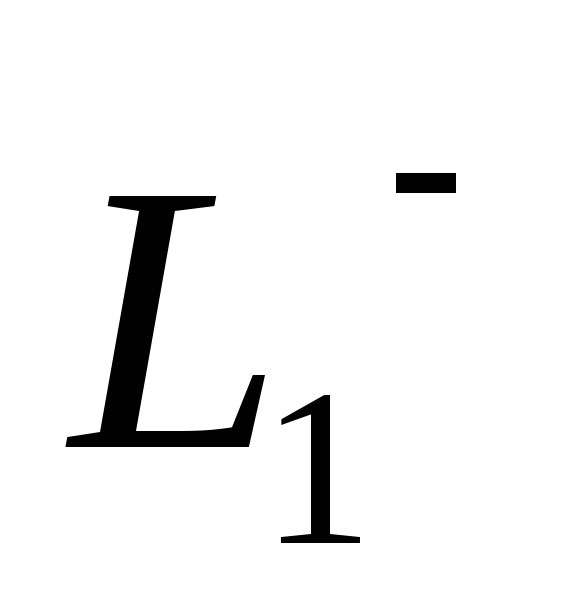be the set of sellers inwho have not received a bid. If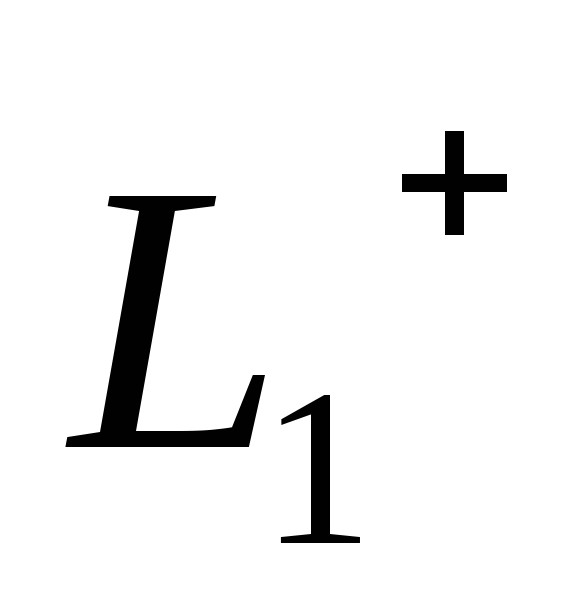is not empty, the buyer should bid with equal probability with every sellers in. Ifis empty butis non-empty, the buyer should bid with equal probability with every seller in. Otherwise, if both subsets are empty, the buyer should bid with equal probability with every seller in. Download 2.41 Mb.Share with your friends: